# The Real Science of the Squat

Why is front squatting more difficult than back squatting when using the same weight? Is the low-bar back squat better for your knees than the high-bar variation? These are all common questions some of us have. In order to answer these questions we have to look behind the curtain of movement and understand the science of squatting.

If you’re a car person, you probably want to know exactly how your engine works. You’ve probably read articles describing the differences between the Chevy Corvette and the Ford Mustang. You understand how horsepower and torque production is different between a turbocharged V6 engine versus a standard V8.

This is your introduction class for the mechanics of the body. We will discuss the differences in torque generations between the squat techniques and what that means for your training. As a word of caution, this article can be a little difficult to comprehend. However, we will do our best to teach these concepts as simply as possible. Welcome to Squat Biomechanics 101.

## Squat Biomechanics 101

The term biomechanics simply refers to the study of forces and how they act on the human body. Biomechanics is the science of breaking down the way we move.

When sport scientists analyze athletes, they often investigate the different forces that are produced during movement. Torque is one of the different parameters that are studied. Torque is the force that causes rotation around a joint.

To explain what torque is and how it affects our body I like to use a simple illustration that I first learned in my college physics class. Many strength and conditioning professionals have used similar examples in their teachings. In particular Mark Rippitoe’s work in his book Starting Strength along with the research from professor Andrew Fry are two great examples that are worth reading (2,3).

Try holding a dumbbell in front of yourself at shoulder height. Do you feel the weight of the dumbbell trying to pull your arm down? What you’re feeling is the force of gravity. It always pulls straight down. As gravity pulls down on the dumbbell, it causes a rotational force at the shoulder joint. This force is torque. The muscles of the shoulder must then be activated to overcome this force in order to hold the weight from moving.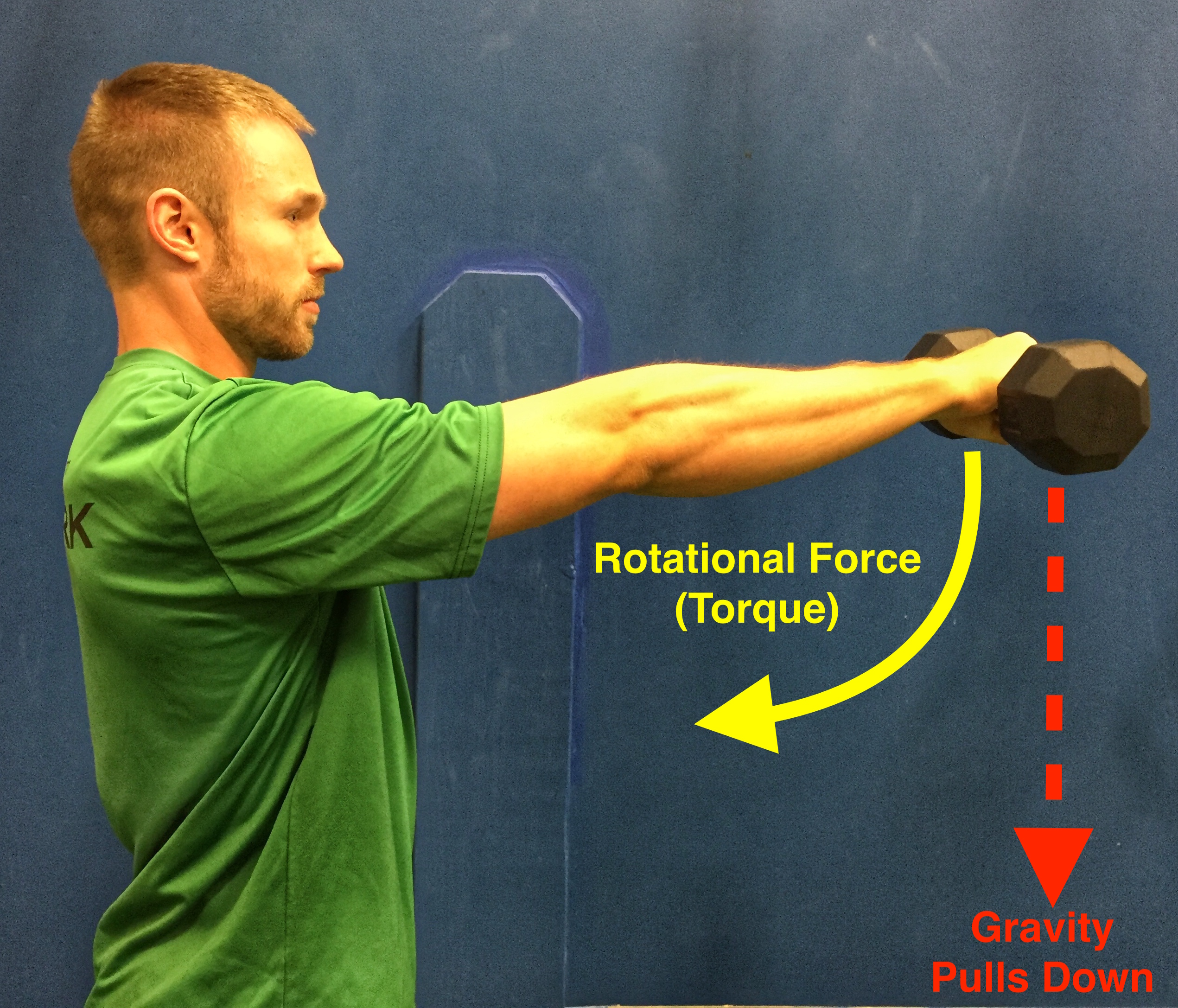In order to calculate how much torque is generated at the shoulder we need to know a few things. First we need to find the length of the persons arm holding the weight. This length between the point of rotation (the shoulder in this case) and the line of force acting upon that joint (the pull of gravity) creates what we call a lever arm.

You can also think of the lever arm as a wrench turning a bolt. When the wrench is pulled down it creates the rotational force torque that turns the bolt.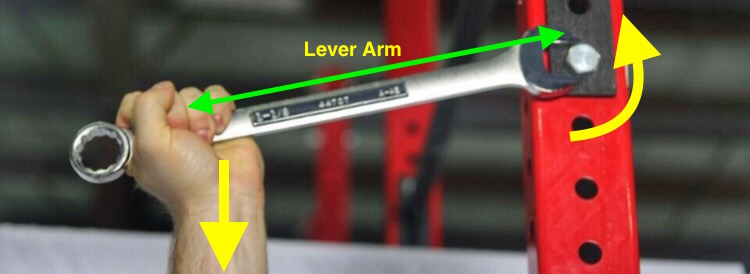Lets take a trip back to physics class and discover how we can calculate this rotational force at a joint. A simple equation to write down is:You’ll notice the word moment arm in the equation instead of lever arm. The moment arm is the perpendicular distance from the start of the lever arm (joint axis) to the vertical force of gravity. It always runs at 90°. For this reason, it will change in length based on the angle the lever arm is held.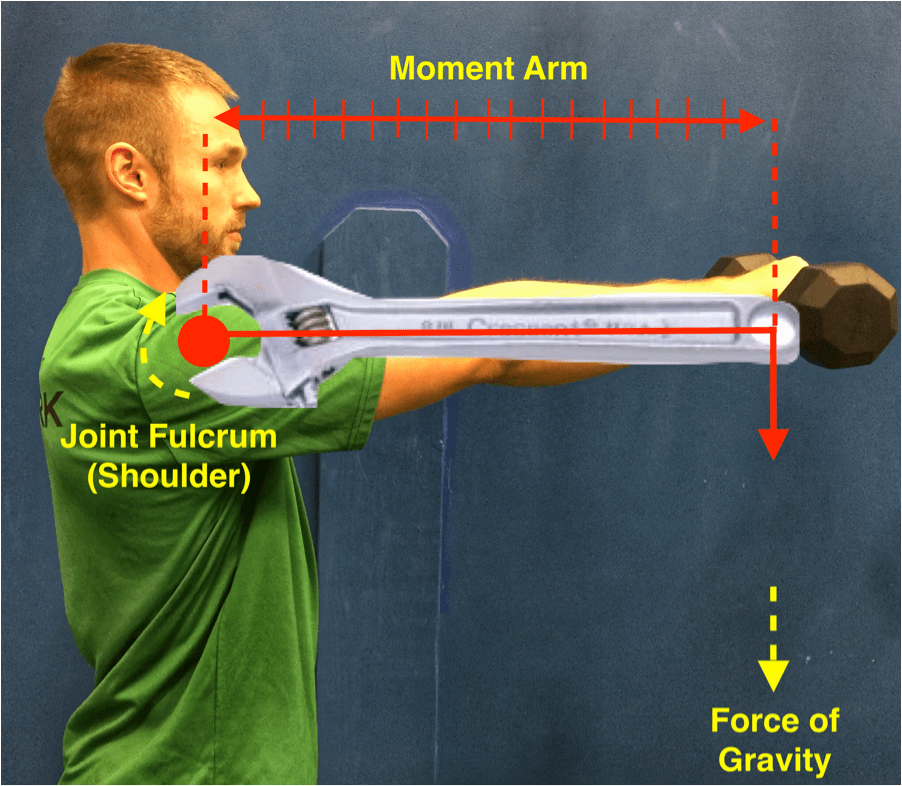In our example the arm is being held straight out in front of the body. This means the arm is already perpendicular to the vertical force of gravity. For this reason the length of our arm (lever arm) will be the exact length of the moment arm. Lets assume your arm is about 75 cm in length (roughly 30 inches). Yes, most mathematical equations also use the metric system.

In order to calculate torque we also need to know how much force is acting on the lever arm. Let’s assume the dumbbell weighs 10 lbs, now convert that 10 lbs to 44.5 Newtons (the unit for force). To get 44.5 Newtons you must convert 10 lbs to 4.54 kg. This is then multiplied by 9.8 m/s2 (standard gravity acceleration) to end up with 44.5 Newtons. A heavier weight would therefore lead to more Newton’s of force.

The equation for torque at the shoulder would look something like this.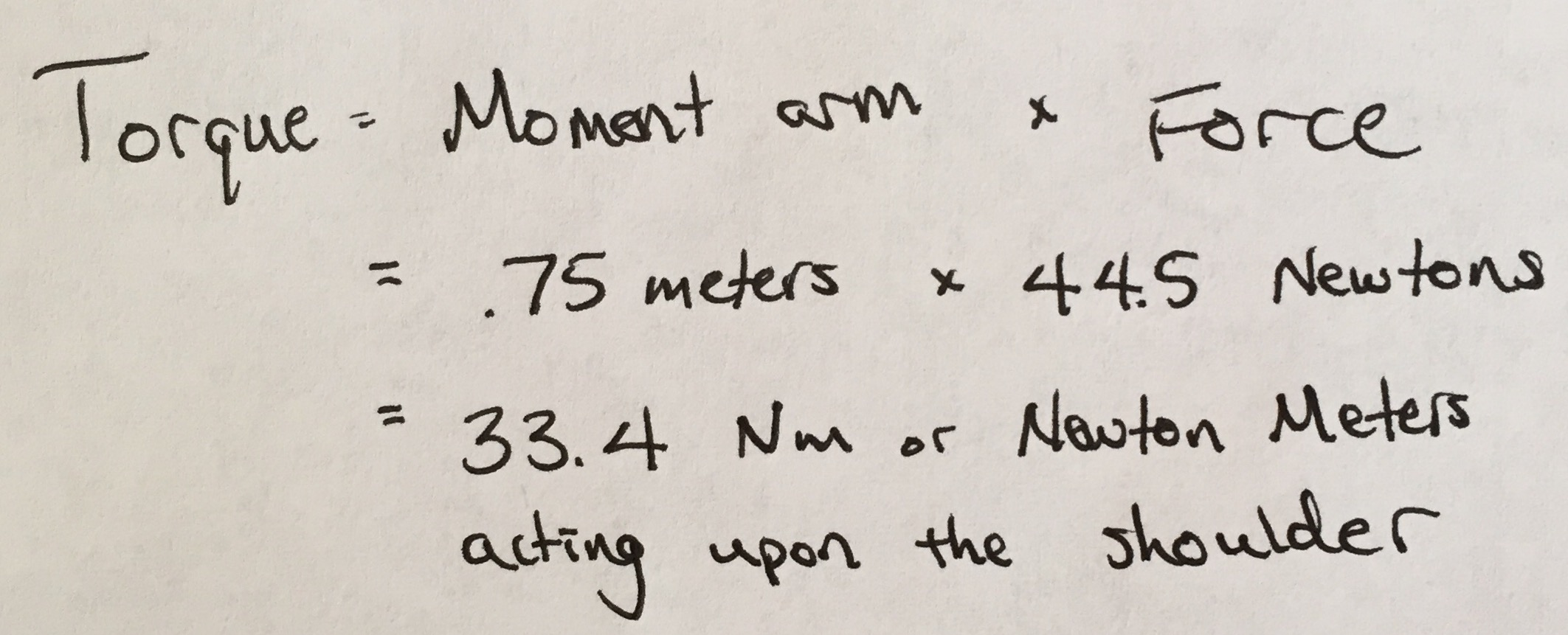This means the muscles of our shoulder need to overcome 33.4 Nm of force (roughly 24.6 foot-pounds of force) to lift the 10-pound weight past the extended position straight out from the body.

You may be asking yourself, “What happens if I raise my arm to a different position?” If we raise the dumbbell above our shoulder joint, we change the length of the moment arm. This is because the arm is no longer perpendicular to the vertical force of gravity. While the length of our arm (the lever) is still the same, the moment arm is now shorter than when our arm was extended straight in front of us.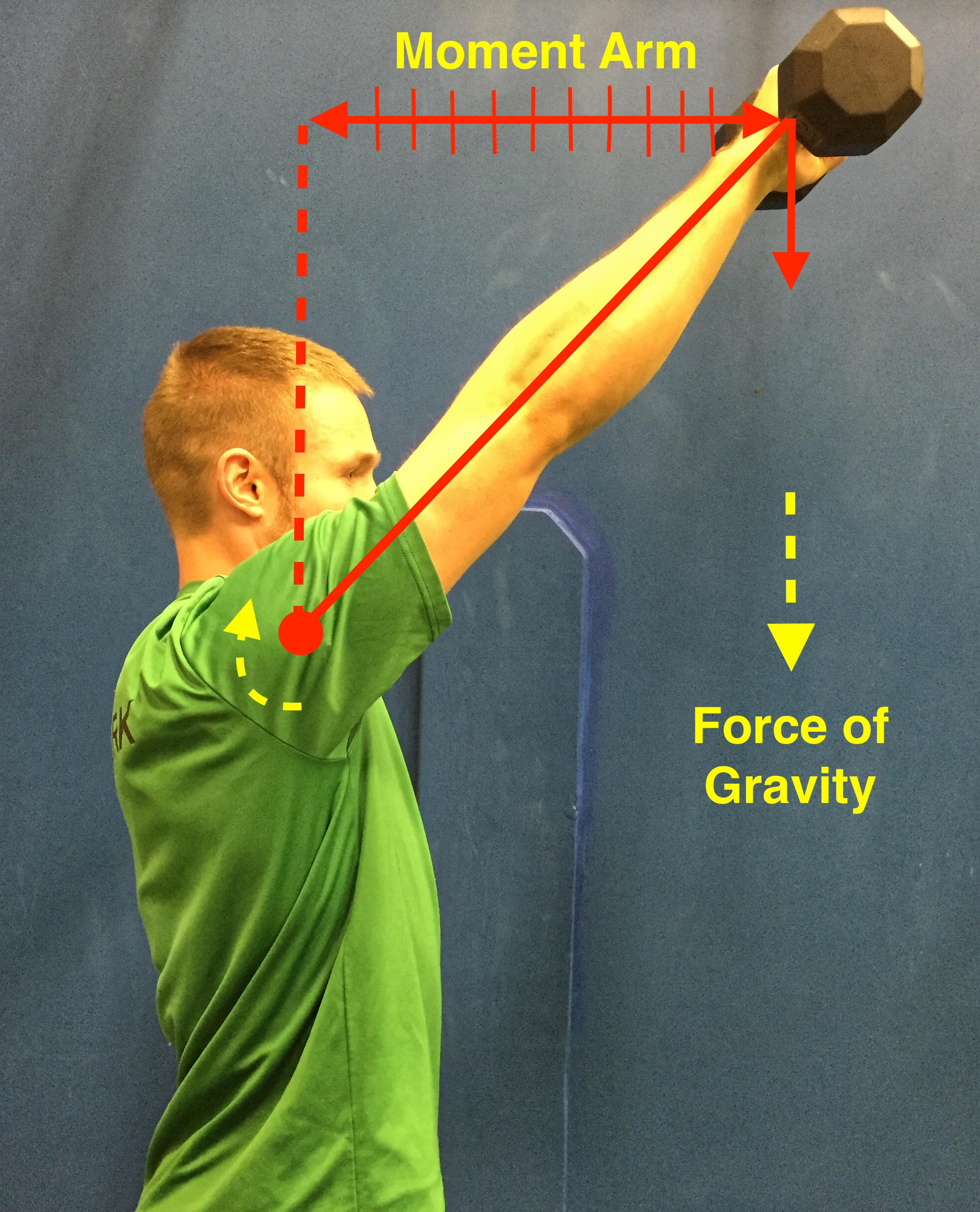This decrease in moment arm length changes the torque placed on the shoulder joint. Lets assume we lifted the arm to an angle of 130°. Because we don’t know the new moment arm length, we need to use trigonometry to calculate this distance. The equation for torque at the shoulder would look something like this.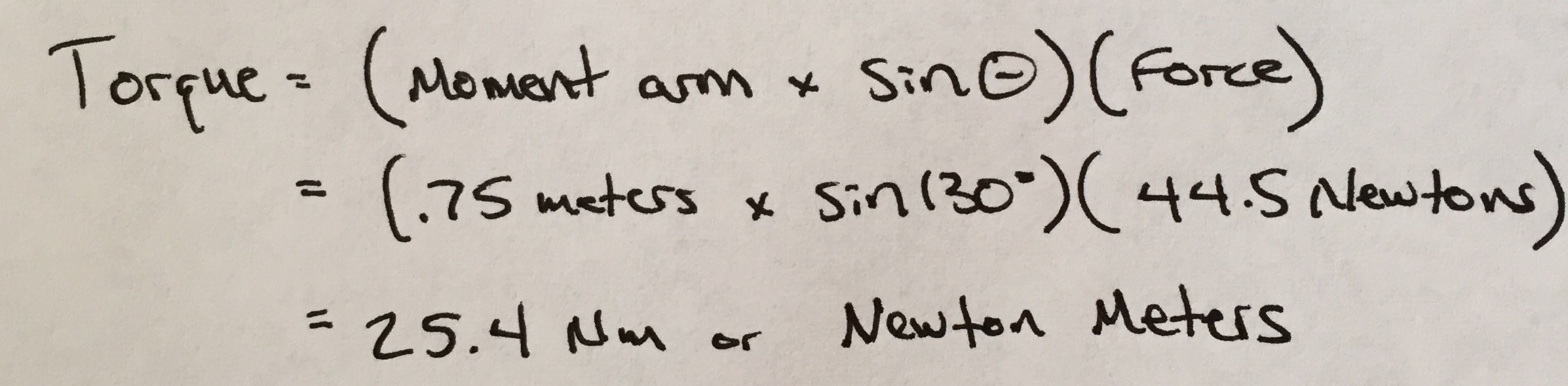When the arm is raised to the higher position, the moment arm becomes shorter. The dumbbell is creating less torque to the shoulder joint. This is why it’s easier to hold the dumbbell close to your chest rather than straight out in front of you.

Another easy way to understand this concept is to perform a slow forward punch with the dumbbell. Is it harder or easier to hold the dumbbell away from your body? Obviously the weight is easier to hold when it’s close to your body! That’s because the moment arm (from weight to shoulder joint) is shorter in this position. A small moment arm generates less torque on a joint when lifting a weight.

## Mechanics of the Squat

When we look at the squat, there are typically three main areas we look at:

1. The knee joint
2. The hip joint
3. The low back

There are two things we need to know when trying to calculate the forces at these joints during the squat. First we need to know the position or angle of the joints. To measure torque, a ‘freeze frame’ or snapshot of the moving body is often taken. This allows us to calculate how much torque is being generated at a specific moment in time. This is called a static model (2).

While the static model for determining joint torques isn’t perfect, most experts suggest it still yields results within 10% of true torque values (3).

When the squat is paused in a certain position, we can then measure the angle of the joints. The back angle is formed by imaginary connection between the trunk and the floor. The hip angle is formed by the position of the back and the thigh. The knee angle is formed by the thigh and the position of the lower leg.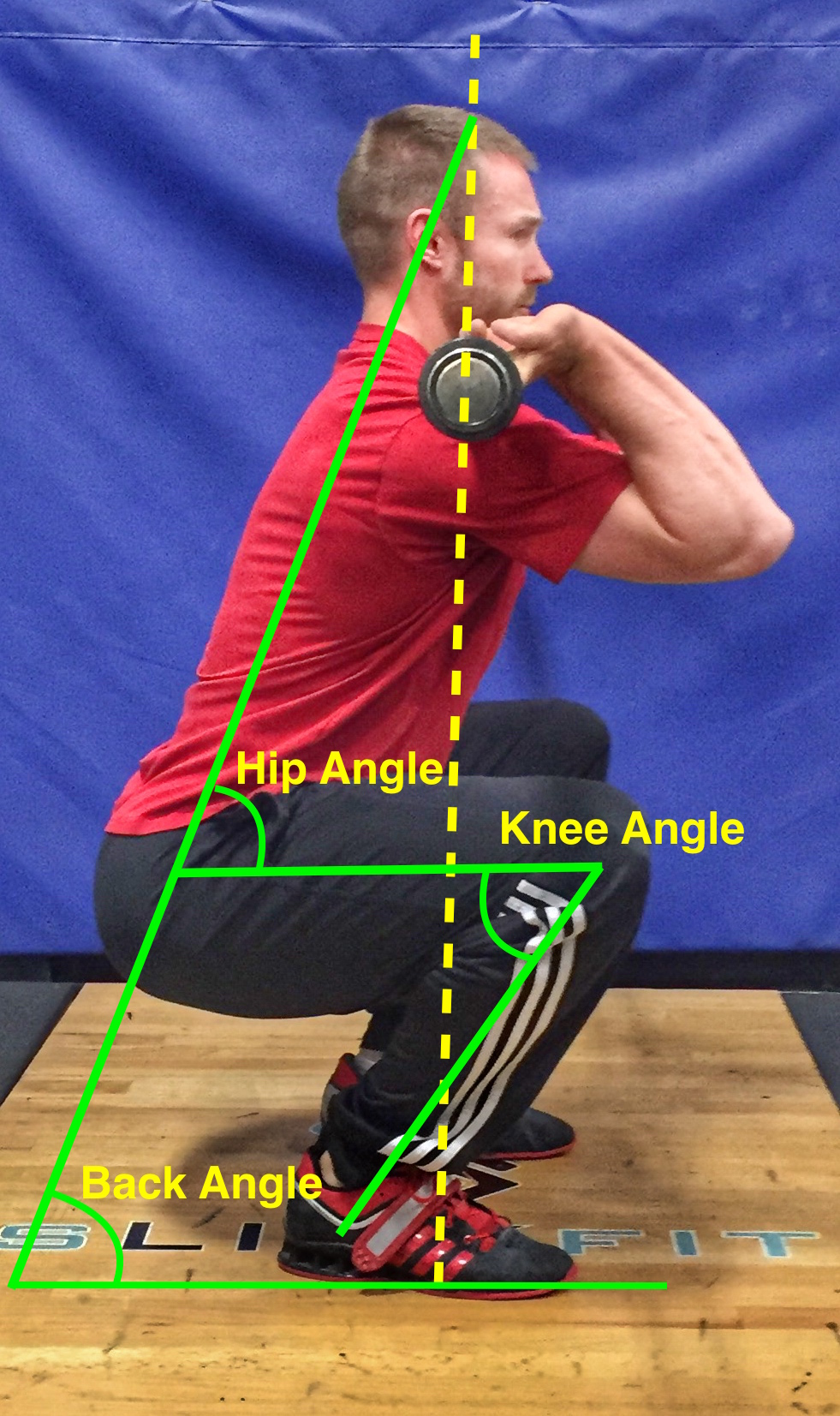Breakout Tip: The knee angle is measured at the point of rotation (knee joint). When the leg is straight the knee is in 0° of flexion. As the knee moves into a flexed position (like when we squat) the angle increases. This is why a deep squat position will be recorded as a knee angle of >120° instead of 60°.Next, we need to measure the length of the lever arms. These distances will change based on the anatomy of the athlete and what kind of barbell squat technique they are performing.

During the squat, gravity pulls down on the barbell just as it did with the dumbbell from our previous illustration. Gravity is often represented as a vertical line drawn through the middle of the barbell. This vertical line then runs through the body and divides the thigh.

During the squat the barbell should track vertically over the middle of an athletes foot. We can use this imaginary line to represent the vertical pull of gravity.

The distance from this vertical line to the center of the joint becomes a lever. Just like the wrench turning the bolt, the length of the lever arm can help us determine the length of the moment arm (1). The longer the moment arm, the more torque that will be generated at that joint during the squat.

Often sport scientists will analyze the squat at a parallel squat position (hip crease in line with the knee) (2,4). At this position (just like the athlete holding the dumbbell directly in front of the body) the lever arm and moment arm will be the same length.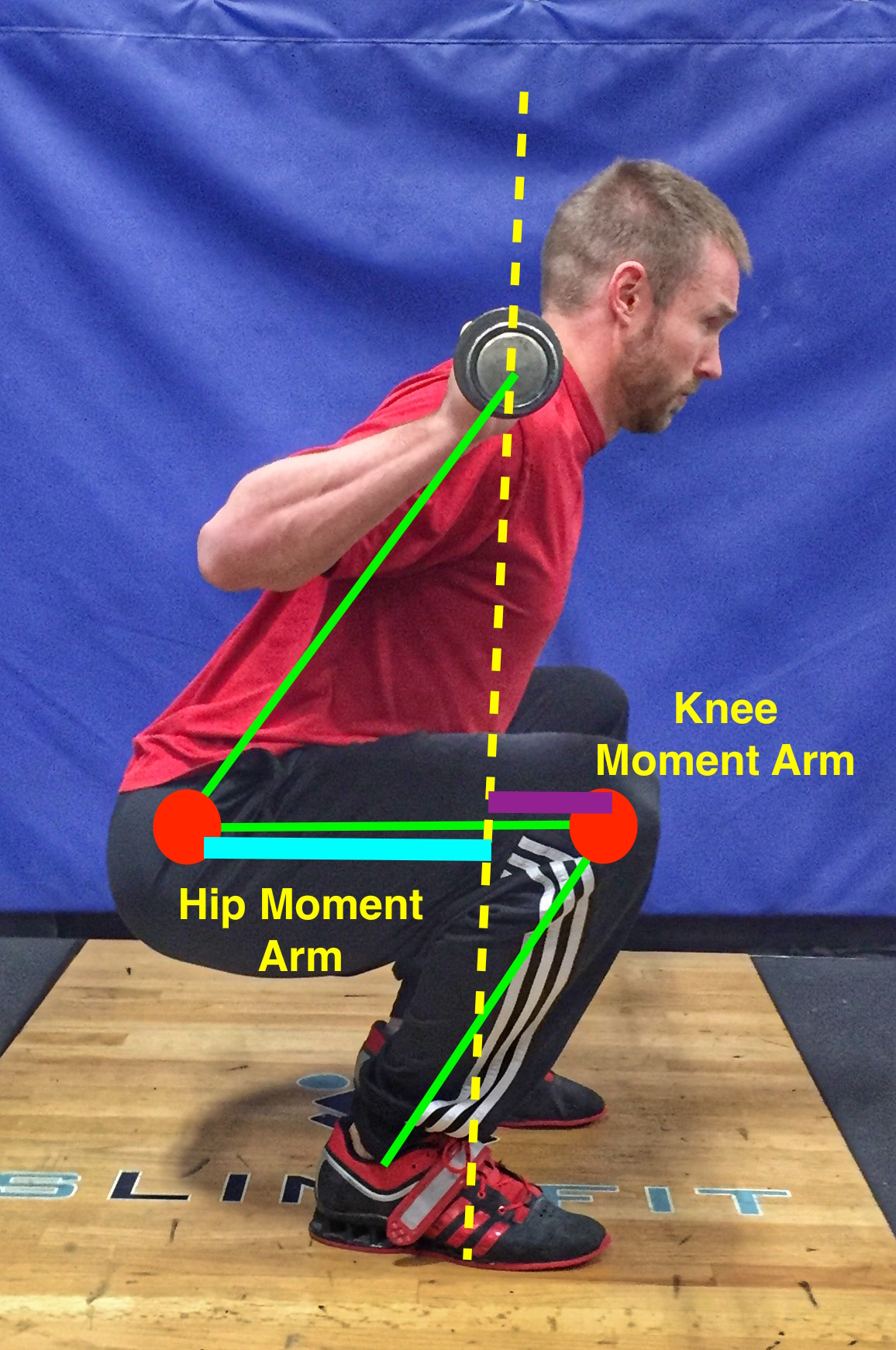## High-Bar Back Squat Analysis (225 lbs)

Lets say we have an athlete squat 225 lbs (102 kg) with a high-bar back squat technique. This technique places the bar on top of the shoulders and upper trapezius muscles near the base of the neck. It is commonly used by weightlifters as it closely mimics the positions used in the competition lifts of the snatch and clean.

At the parallel position of this squat we can “freeze frame” the movement. For this illustration lets say the knee ends up at an angle of 125° and the angle the hip is 55°. The back angle would also be 55°. Since we are assuming a parallel thigh position to the floor, the hip angle and back angle will be the same.

In order to simplify this analysis (and save ourselves some difficult trigonometry) we’re going to measure the moment arms. Assume the knee moment arm in this high-bar back squat is 7.5 inches long (or .19 meters for mathematical purposes) and the hip moment arm that is 10.5 inches long (or .27 meters). Remember the moment arm length is the perpendicular distance from the joint to the vertical line of gravity that runs through the middle of the leg. This means the overall thigh length is 18 inches long (hip lever arm + knee lever arm = full thigh length).

For the purposes of this analysis, the low back will be represented as the connection of the spine to the pelvis. For this reason the moment arm will be the distance from this point to the vertical line of gravity. Because this axis of rotation is relatively close to the hip joint, the back lever arm will be the exact same distance as the hip lever arm (1)

In order to do this calculation we also need to figure in the weight of the barbell so we know how much force is pulling down. The weight of 225 lbs is equal to 1000.85 Newton’s of force. We can now plug these numbers into our mathematical equation to determine torque.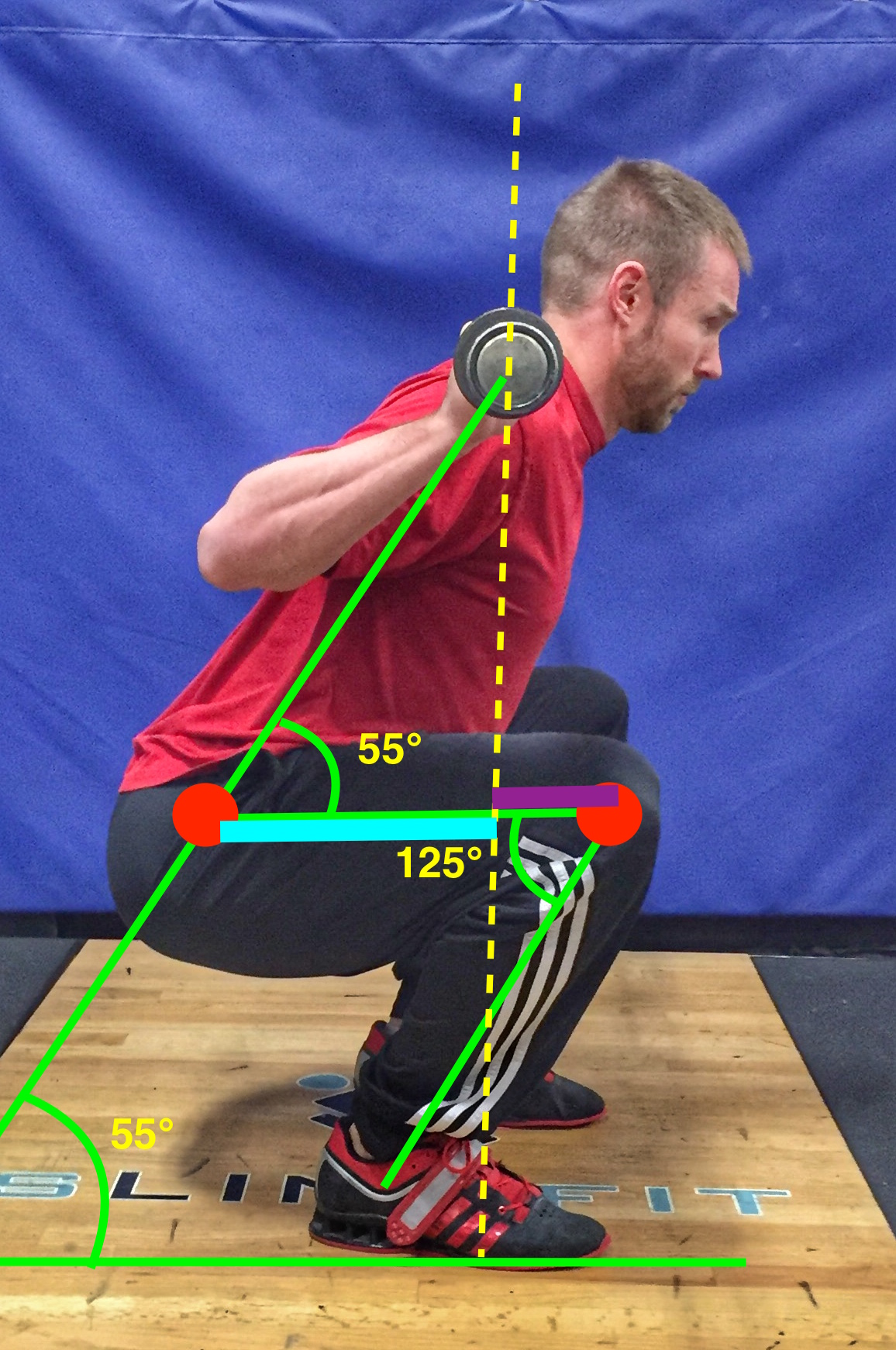## Low-Bar Back Squat Analysis (225 lbs)

What if this same athlete now squatted 225 lbs with a different technique? Lets assume this athlete is now lifting with a low-bar back squat technique. This variation uses a bar position that is 2-3 inches lower on the back than the high-bar back squat technique. The bar commonly rests in the middle of the shoulder blade. Powerlifters commonly use it as it enables them to lift heavier weights (5). In order to maintain balance (bar positioned over the middle of the foot) the chest must learn forward to a greater degree (6).

Doing so does two things to the mechanical levers of the body. First, the forward lean of the trunk drives the hips backwards. This lengthens the hip and back moment arm. It also shortens the knee moment arm.

Lets assume the knee moment arm is now 5.5 inches (.14 meters) compared to the 7.5 inches during the high-bar technique. This would obviously lengthen the hip moment arm from 10.5 inches to 12.5 inches (.32 meters)

At the parallel “freeze-frame” position we see this lifter assuming slightly different position:

• Knee angle of 120° (larger or more open than the high-bar technique)
• Hip and back angle of 40° (a smaller or more closed angle than the high-bar technique due to the more inclined chest position)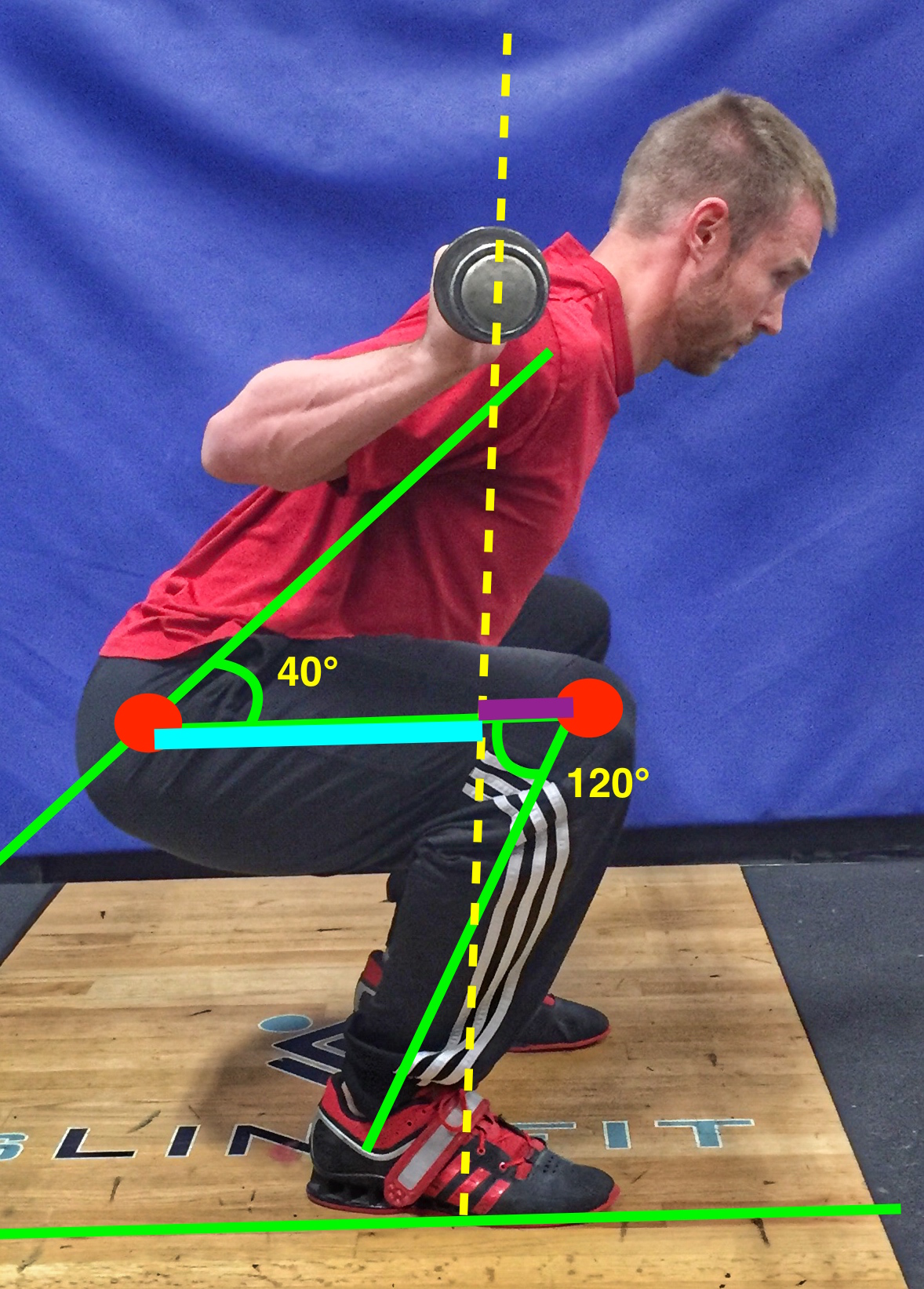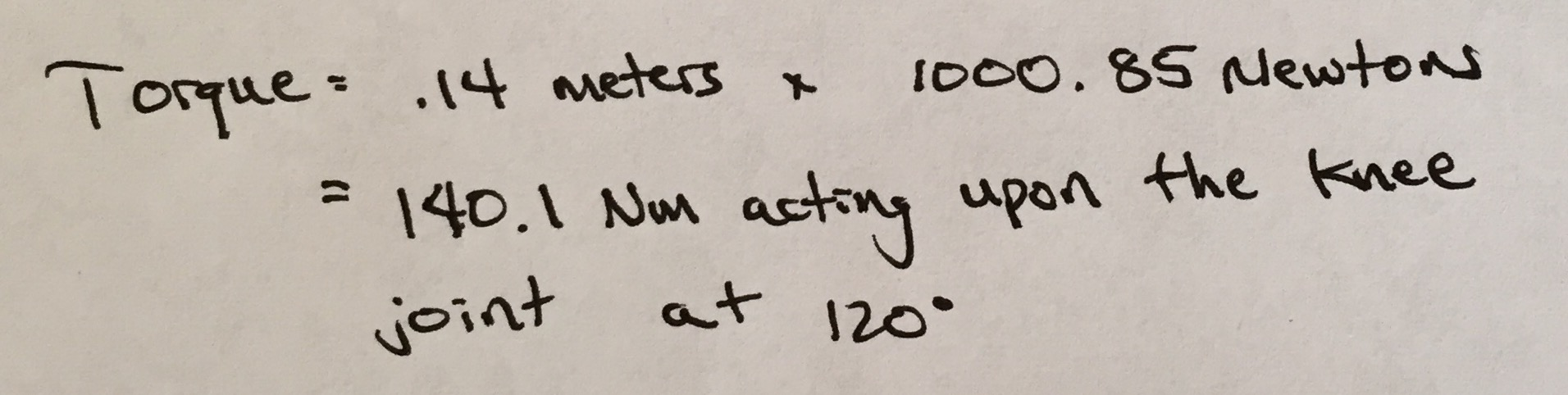## Front Squat Analysis (225 lbs)

Lets now look at the front squat. The front squat loads the joints differently than the previous two techniques. This is because the bar is held on the chest. This will require a more vertical trunk position in order to keep the bar positioned over the middle of the foot and allow the body to remain in balance. This lift is also used often by weightlifters as the movement closely mimics the clean movement.

The hips and knees will inevitably be pushed forward in order to maintain balance because the trunk must be held in a more upright position. If you tried to front squat and push your hips back too far, the bar will likely roll of your chest and end up on the ground.

Lets assume the athlete’s knee moment arm length is now 8.5 inches (.22 meters). This is longer than the high-bar back squat. This is a common change as the knee frequently translates a bit further forward in the front squat in order to remain in balance. This longer knee moment arm then creates a shorter hip moment arm, now measured at 9.5 inches (.24 meters).

If we “freeze-frame” the front squat in the parallel thigh position, we see a few differences compared to the other squats.

• Knee angle of 130° (smaller or more closed compared to both back squat techniques due to the more forward knee position)
• Hip and back angles of 75° (larger or more open compared to the back squat techniques due to the more upright chest position)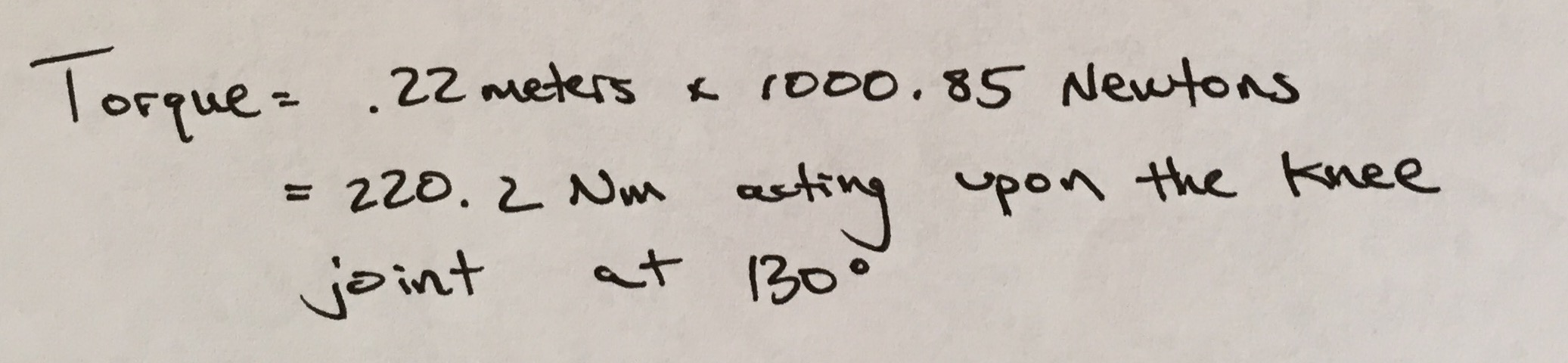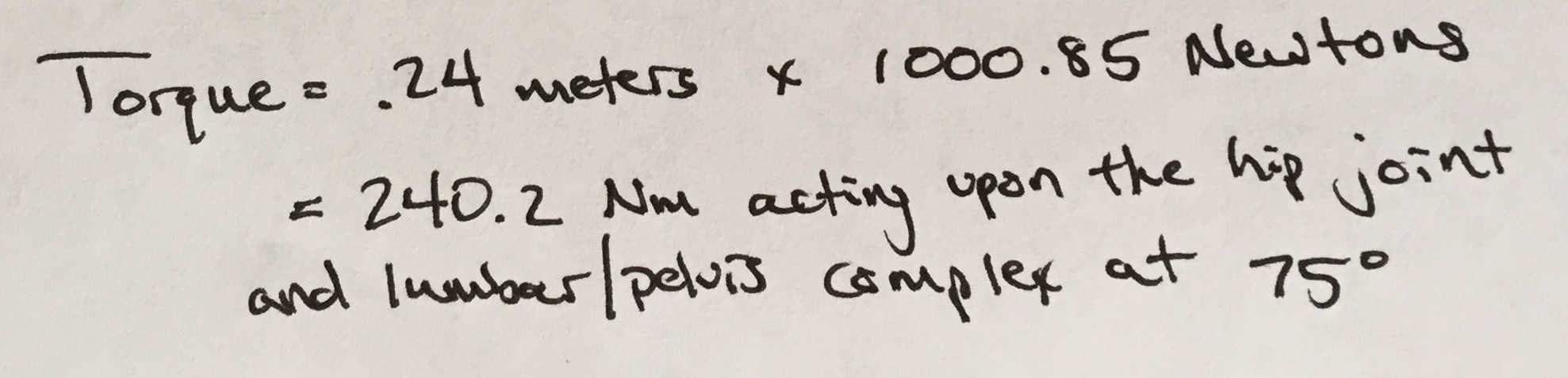## Comparative Analysis (225 lbs)

In this article we assessed an athlete lifting a barbell loaded to 225lbs (102 kg) with three squat technique variations. After calculating torque at the same depth across all three squats we are able to see a few interesting things:

• The front squat placed the most amount of torque on the knee joint (220.2 Nm) followed closely by the high-bar back squat (190.2 Nm) and then by the low-bar back squat (140.1 Nm). This means the front squat placed roughly 15% more torque on the knees than the high-bar squat and 57% more than the low-bar squat.
• The front squat placed less torque on the hip and lower back (240.2 Nm at the lumbar/pelvis connection) compared to both back squat techniques (high-bar 270 Nm and low-bar 320.3 Nm). This means the front squat placed 12% less torque on the hip than the high-bar back squat and 25% less than the low-bar back squat.

If an athlete lifts the same weight with all three squat techniques, we can assume the front squat will be the most difficult to perform. According to this analysis, the low-bar back squat would be the easiest and most efficient way to lift the 225 lbs. The low-bar back squat is the most mechanically efficient technique. It all comes down to leverage. Mechanically, our bodies can squat more weight when the moment arm is longest at the hips (5).

Many experienced lifters will agree that it’s easier to lift more weight with the back squat technique when compared to the front squat. Also, when watching a powerlifting meet, almost all of the lifters will use a low-bar back squat to compete and not the high-bar squat.

Next week, we will take a more in-depth look on torque and the 3 different squat techniques.

Until next time,

With

Resources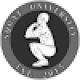## 15 thoughts on “The Real Science of the Squat”

1. Outstanding in-depth description here. I will have to go back through this again to pack in all the info. This is a super unique post as I don’t usually see such informative and scientific posts. I love it though. Low bar is the way to go! Keep it up guys!

1. Thank you! Glad you like the post!

2. Why do you use sine instead of cosine for the torque equation when the moment arm is adjacent to, not opposite from, the joint angle?

1. Eric, we made some changes to our math. Thanks for the find!

3. […] #5: The Real Science of The Squat […]

4. […] of other lifts including the low-bar back squat. This variation allows athletes to manipulate joint torque production in order to lift more weight than any other squat technique. By sitting the hips back to […]

5. […] year ago I wrote a blog article entitled “The Real Science of the Squat” where I broke down the biomechanics of the different squat techniques. In the article I explained […]

6. interesting

7. I just love to play sports and I read articles here very often. Unfortunately, I could not practice sports often because I had problems with my studies. By the way, I ordered paperell review here EssayServiceScanner and got a great review. I continue to order work there, so I can do sports.

8. Where’s the follow up post

9. You have made the bio mechanics of squat crystal clear !

10. […] The front squat clearly brings the center of gravity further forward relative to the back squat, and therefore does a better job of recruiting the quadriceps. […]

11. […] #5: The Real Science of The Squat […]

12. […] back to Squat University! Last week we entered into Biomechanics 101, an introduction into the mechanics of the human body. We learned what torque is and how it is […]